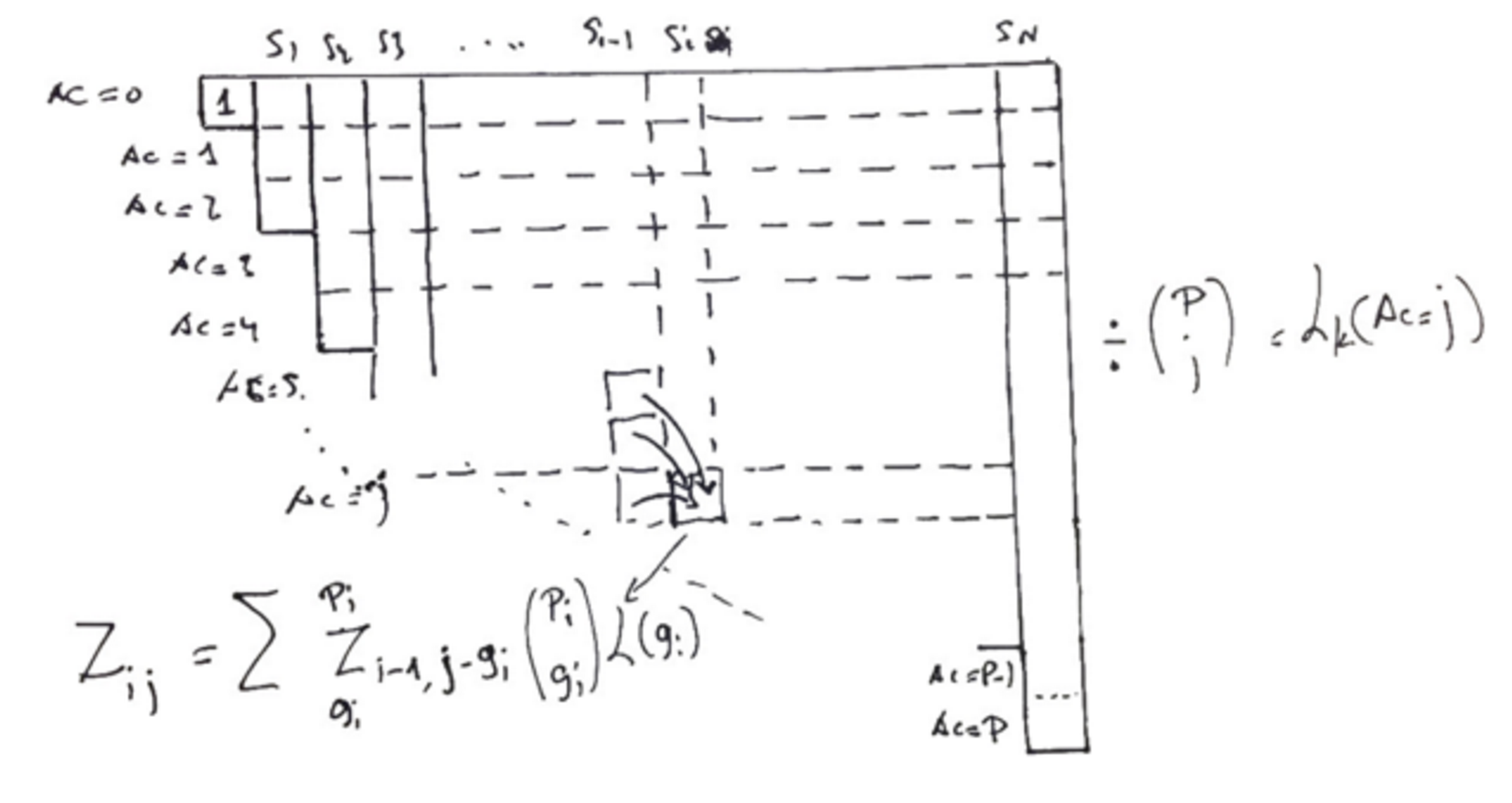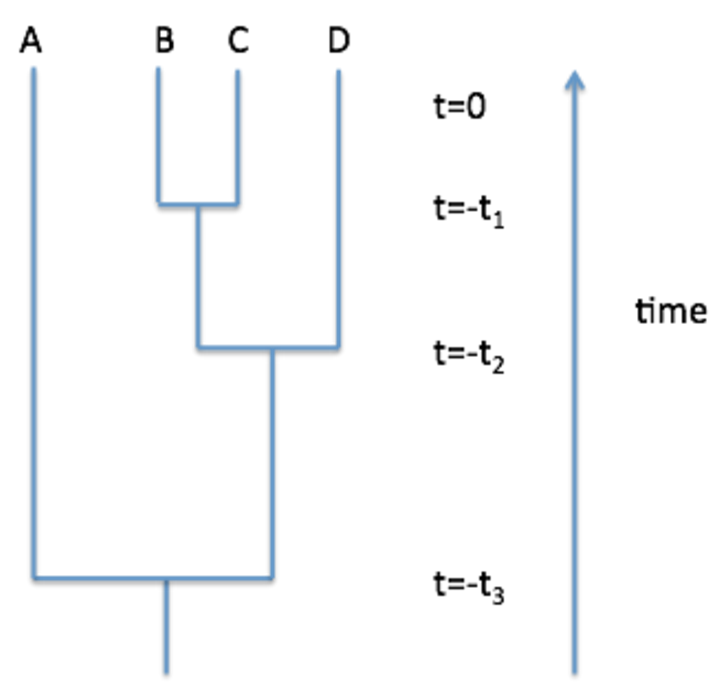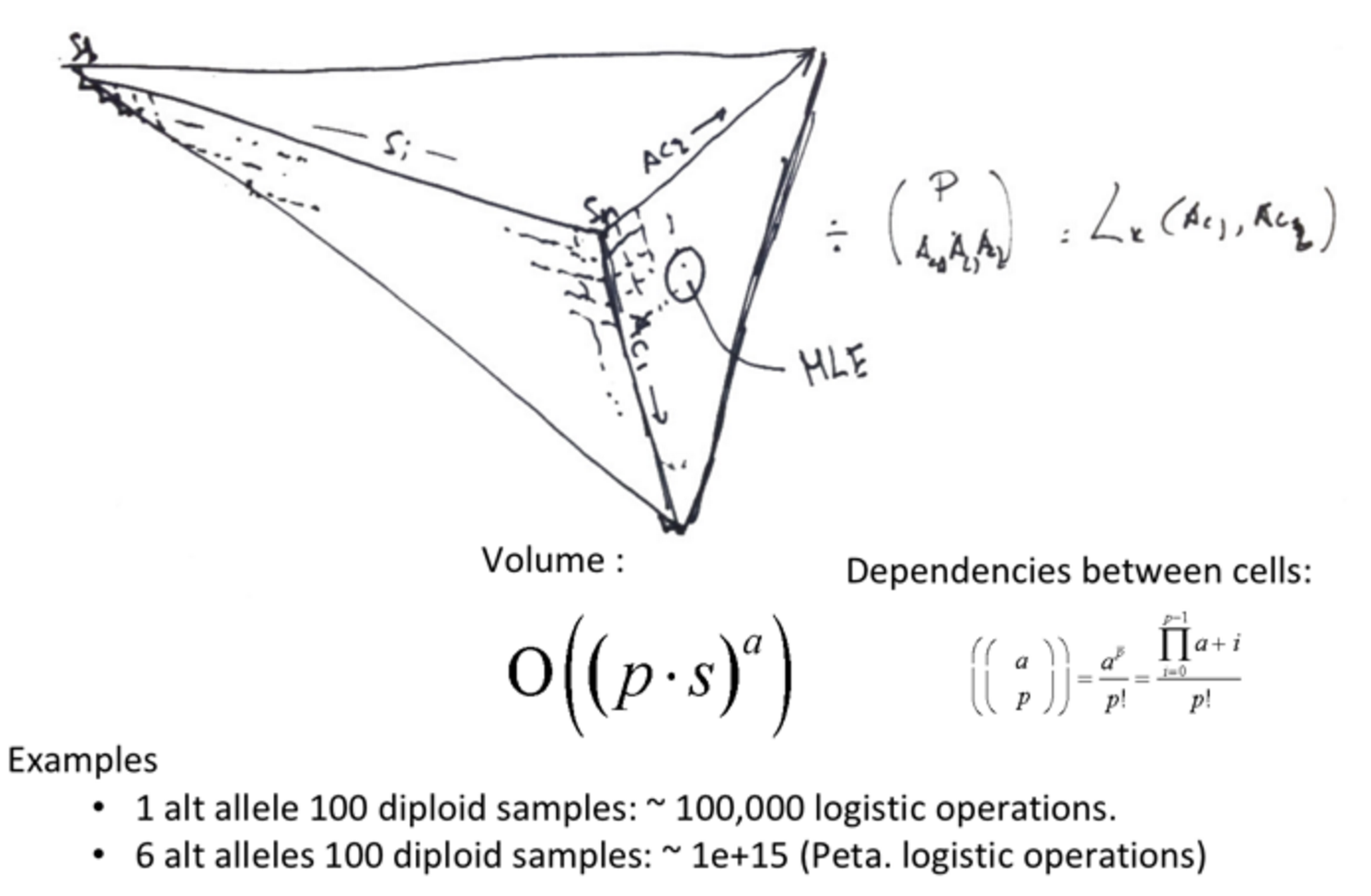We've moved!
This site is now read-only. You can find our new documentation site and support forum for posting questions here.
Be sure to read our welcome blog!

# Math notes: Understanding the QUAL score and its limitations

edited May 2016

It used to be that the first rule of GATK was: don't talk about the QUAL score. No more! This document covers the key points in loving detail. Figures are hand-drawn and scanned for now; we'll try to redo them cleanly when we find a bit of time (don't hold your breath though).

### What is the QUAL score?

It's the Phred-scaled posterior probability that all samples in your callset are homozygous reference.

### Okay, but really, what does it tell us?

Basically, we're trying to give you the probability that all the variant evidence you saw in your data is wrong.

If you have just a handful of low quality reads, your QUAL will be pretty low. Possibly too low to emit -- we typically use a threshold of 10 to emit, 30 to call in genotyping, either via HaplotypeCaller in "normal" (non-GVCF) mode or via GenotypeGVCFs (in the GVCF workflow, HaplotyeCaller sets both emit and call thresholds to 0 and emits everything to the GVCF).

However, if you have a lot of variant reads, your QUAL will be much higher. But it only describes the probability that all of your data is erroneous, so it has trouble distinguishing between a small number of reads with high quality mismatches or a large number of reads with low quality mismatches. That's why we recommend using QualByDepth (the QUAL normalized by depth of reads supporting the variant) as an annotation for VQSR because that will yield higher annotation values for high quality reads and lower values for big piles of weak evidence.

### I know the PLs give the genotype likelihoods for each sample, but how do we combine them for all samples?

Heng Li's 2011 paper, section 2.3.5 (there are other copies elsewhere) gives the equations for the biallelic case. It's a recursive relation, so we have to use a dynamic programming algorithm (as you may have seen in the chapter on pairwise alignments in the Durbin et al. "Biological Sequence Analysis" book).

This lovely diagram lays it all out:S_1...S_N are your N samples, which we're going to evaluate cumulatively as we move across the columns of the matrix. Here we're being very general and allowing each sample to have a different ploidy, which we'll represent with p_i. Thus the total number of chromosomes is Sum{p_i}=P.

We're interested in the S_N column because that represents the AC calculations once we take into account all N samples. The S_N column still isn't our final joint likelihood because we added the samples in a particular order, but more on that later.

We calculate the joint likelihood across samples for all ACs from zero to the total number of chromosomes. We look at all ACs because we also use this calculation to determine the MLEAC that gets reported as part of the "genotyping" process. In the matrix above, we're indexing by i for sample and j for allele count (AC). g_i represents the genotype of the ith sample in terms of its number of alt alleles, i.e. for homRef g_i=0. Note that uses a different approach to break things down than Heng Li's paper, but it's more intuitive with the indexing. And remember this is the biallelic case, so we can assume any non-reference alleles are the same single alt allele. L(g_i) is the likelihood of the genotype, which we can get from sample i's PLs (after we un-Phred scale them, that is).

The "matrix" is triangular because as AC increases, we have to allocate a certain number of samples as being homozygous variant, so those have g_i = 2 with probability 1. Here we show the calculation to fill in the z_ij cell in the matrix, which is the cell corresponding to seeing j alt alleles after taking into account i samples. If sample i is diploid, there are three cells we need to take into account (because i can have 3 genotypes -- 0/0, 0/1, and 1/1 corresponding to g_i={0,1,2}), all of which come from the column where we looked at i-1 samples.

Thus z_ij is the sum of entries where i-1 samples had j alts (z_i-1,j,and sample i is homRef), where i-1 samples had j-1 alts (z_i-1,j-1 and sample i is het) and where i=1 samples had j-2 alts (z_i-1,j-2 and sample i is homVar), taking into account the binomial coefficient (binomial because we're biallelic here so we're only interested in the ref set and the alt set) for the number of ways to arrange i's chromosomes.

By the time we get to column S_N, we've accumulated all the PL data for all the samples. We can then get the likelihood that AC=j in our callset by using the entry in the row according to AC=j and dividing it by the binomial coefficient for the total number of chromosomes (P) with j alt alleles to account for the fact that we could see those alt chromosomes in our samples in any order.

### Wait, that's just a likelihood. But you said that the QUAL is a posterior? So that means there's a prior?

Yep! In short, the prior based on AC is Pr(AC = i; i > 0) = θ/i making Pr(AC = 0) = 1 – ΣP>=i>0Pr(AC = i)

### What's the long version?

The prior, which is uniform across all sites, comes from population genetics theory, specifically coalescent theory. Let's start by defining some of our population genetics terminology. In the GATK docs, we use θ as the population heterozygosity under the neutral mutation model. Heterozygosity is the probability that two alleles drawn at random from the population will be different by state. In modern sequencing terms, that just means that there will be a variant in one with respect to the other. Note that two alleles can be identical by state but different by origin, i.e. the same variant occurred independently. If we assume that all loci are equally likely to be variant (which we know in modern times to be false, but this assumption underlies some important population genetics theories that we us as approximations) then we can also describe θ as the rate at which variants occur in the genome, 1 per 1/θ basepairs.

From Gillespie, "a coalescent is the lineage of alleles in a sample [as in cohort of individuals samples from the population] traced backwards in time to their common ancestor allele." Forward in time, the splits in the tree can be thought of as mutation events that generate new alleles. Backwards in time, they are referred to as coalescent events because two branches of the tree coalesce, or come together.Each split in the coalescent represents the occurrence of a variant (let's say that each left branch is unchanged and the right branch picks up the new variant). Allele A never saw any variants, but one occurred separating A from B/C/D at -t3. Then another occurred separating B/C from D at -t2, and a final one separating B from C at -t1. So allele A is still "wild type" with no variants. Allele B has only variant -t3. Allele C has two variants: t3 and t1. Allele D has two variants: t3 and t2. So variant t3 has AC=3 (three alleles stemming from its right, non-reference branch), t2 has AC=1 and t1 has AC=1. Time here is given in generations of the population, so multiple generations can occur without there being a mutational event leading to a new allele.

The total time in the coalescent is measured as the sum of the lengths of all the branches of the tree describing the coalescent. For the figure, Tc = 4t1 + 3(t2-t1) + 2*(t3-t2). If we define Ti as the time required to reduce a coalescent with i alleles to one with i-1 alleles, we can write Tc as 4T4 + 3T3 + 2T2. In the forward direction, then Ti becomes the amount of time required to introduce a new mutation into a population of i-1 distinct alleles.

To derive an expected value for Ti, let's look at how each allele is derived from its ancestors in a population of n alleles within N samples under the assumption that a coalescence has not occurred, i.e. that each allele has a different ancestor in the previous generation because there were no coalescence events (or mutations in the forward time direction). The first (reference) allele (A in the diagram) is guaranteed to have an ancestor in the first generation because there were no mutations. The second allele has to have a different ancestor than the first allele or else they would be derived from the same source and thusly the same allele because there were no mutations in this generation. The second allele has a different ancestor with probability 1-1/(2N) = (2N-1)/(2N) (where we're assuming ploidy=2 as we usually do for population genetics of humans). Note that there are 2N possible ancestor alleles and 2N-1 that are different from the first allele. The probability that the third allele has a distinct ancestor, given that the first two do not share an ancestor, is (2N-2)/(2N), making the total probability of three alleles with three different ancestors:

$$\dfrac{2N-1}{2N} \times \dfrac{2N-2}{2N}$$

We can continue this pattern for all n alleles to arrive at the probability that all n alleles have different ancestors, i.e. that no coalescent event (or variant event) has occurred:

$$\left ( 1-\dfrac{1}{2N} \right )\times \left ( 1-\dfrac{2}{2N} \right )\times \cdots \times \left ( 1- \dfrac{n-1}{2N} \right )$$

And if we multiply terms and approximate terms with N^2 in the denominator is small enough to be ignored we arrive at:

$$1- \dfrac{1}{2N}-\dfrac{2}{2N}- \cdots - \dfrac{n-1}{2N}$$

The probability of a coalescence occurring is the complement of the probability that it does not occur, giving:

$$\dfrac {1+2+\cdots+(n-1)}{2N} = \dfrac{n(n-1))}{4N}$$

Which is the probability of a coalescence in any particular generation. We can now describe the probability distribution of the time to the first coalescence as a geometric distribution where the probability of success is:

$$E[T_n] = \dfrac{4N}{n(n-1))}$$

Giving the expectation of the time to coalescence as:

$$E[T_i] = \dfrac{4N}{i(i-1))}$$

We can generalize this to any coalescent event i as:

$$T_c = \sum_{i=2}^{n}iT_i$$

Which is a generalization of the example worked out above based on the figure. The expectation of the time spent in the coalescent is then:

$$E[T_c] = E \left[ \sum_{i=2}^{n}iT_i\right ] = \sum_{i=2}^{n}iE[T_i] = 4N \sum_{i=2}^{n}\dfrac{1}{i-1}$$

The expected number of variants (Sn, called "segregating sites" in the old-school pop gen vernacular) is the neutral mutation rate (u) times the expected amount of time in the coalescent. A mutation can occur on any branch of the coalescent, so we want to sum the time in all branches to allow for all possibilities -- we did this above.

So the expected number of variants can be expressed in terms of the heterozygosity, which, if we describe it as a rate per basepair as above, allows us to describe the probability of a variant occurring at a given locus, forming the prior for our QUAL score. If we assume a cohort of unrelated individuals, the occurrence of any variant with AC > 1 must the result of that variant occurring multiple times independently at the same locus. If we now assume the coalescent is restricted to lineage of variants at a single position, we can reframe E[Sn] in terms of AC instead of number of alleles. Then we can convert the index of the sum to be AC (the number of mutations, but restricted to the same locus) using i = i' + 1 (because the set n originally includes the reference allele) so that the new
where N is the number of chromosomes in the cohort.

From there, we can show that the Pr[AC=i] = θ/i

$$E[S_n] = uE[T_c]=\theta\sum_{i=2}^{n}\dfrac{1}{i-1} = \dfrac{\theta}1+\dfrac{\theta}2+\cdots+\dfrac{\theta}{n-1}$$

(The theory presented here comes from Chapter 2 of "Population Genetics: A Concise Guide" by John H. Gillespie)

### And the final QUAL calculation?

The posterior is simply:

P(AC = i|D) = Lk(D | AC = i) Pr(AC = i) / P(D)

QUAL = Phred ( AC = 0 | D).

### Okay, but biallelic sites are boring. I like working with big callsets and multiallelic sites. How does the math change in that case?

Well, the short answer is that it gets a lot more complicated. Where we had a 2-D matrix for the biallelic case, we'll have a N-dimensional volume for a site with N alleles (including the reference.)

Another lovely illustration helps us wrap our puny human brains around this idea:Where p is ploidy, s is number of samples, a is number of alleles -- that's it.

So we use some approximations in order to get you your results in a reasonable amount of time. Those have been working out pretty well so far, but there are a few cases where they don't do as well, so we're looking into improving our approximations so nobody loses any rare alleles. Stay tuned!

Post edited by Sheila on
Tagged:

• thanks for this post, I'm currently reading through this and stumbled onto something that looks like an inconsistency to me. In the third major section it first says:

g_i represents the genotype of the ith sample in terms of its number of alt alleles, i.e. for homRef g_i=0.

And then one paragraph later, g_i seems to be defined the other way around:

The "matrix" is triangular because as AC increases, we have to allocate a certain number of samples as being homozygous variant, so those have g_i = 0 with probability 1. [...] (because i can have 3 genotypes -- 0/0, 0/1, and 1/1 corresponding to g_i={2,1,0}), all of which come from the column where we looked at i-1 samples.

Best regards,
David

@laehnemann
Hi David,

Thanks for pointing that out. I need to confirm with the team which is the correct definition, then I will fix it.

Thanks,
Sheila

I just fixed it. g_i is indeed the number of alt alleles, so the first paragraph you referenced was correct. Sorry for the confusion. I was going to rephrase some of the paragraphs here, but I was just told the QUAL score calculation will be changing, so that may be more effort than necessary